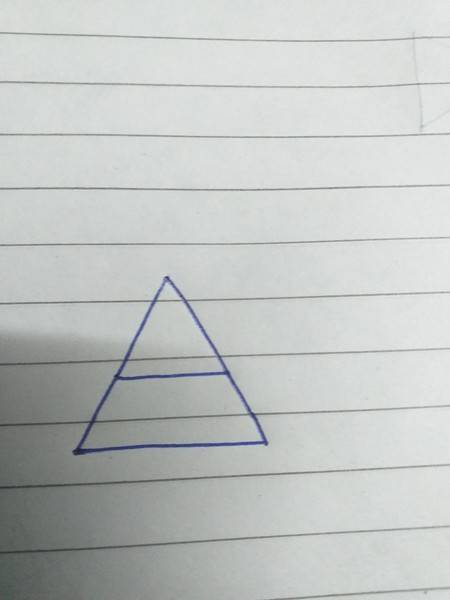# Polygon or not?

• B
Suyogya
Can someone please tell is this (https://ibb.co/stGFSKs) figure a polygon. If yes then is the middle line would count as an edge?#### Attachments

Homework Helper
Yes, it could be described as a polygon - but not a "simple polygon".
https://en.wikipedia.org/wiki/Polygon

If the left edge from top to bottom is A, B, D and the right edge from top to bottom is A, C, E:
Then this could be described as polygon ABCBDECA or ABCEDCDA.

Thus, it would be a polygon that intersects itself.
polygons that do not intersect themselves are called "simple polygons".

Oh, and to answer your follow-on question: The middle line segment would be two edges.

Suyogya
Yes, it could be described as a polygon - but not a "simple polygon".
https://en.wikipedia.org/wiki/Polygon

If the left edge from top to bottom is A, B, D and the right edge from top to bottom is A, C, E:
Then this could be described as polygon ABCBDECA or ABCEDCDA.

Thus, it would be a polygon that intersects itself.
polygons that do not intersect themselves are called "simple polygons".

Oh, and to answer your follow-on question: The middle line segment would be two edges.
let be name the points as,(https://ibb.co/2srLjB1) if AC would be an edge then A and C are vertices and segments BC, AC, AD, CE became edges. Is this justified Also for a simple polygon there should be no self intersecting edge, the line AC just touches the lines BD and BE, is this sufficient to fulfill the intersecting condition?

Homework Helper
In order for it to be a polygon, you need to close the loop - end at the same place you started.
I am not sure I am interpreting your statements correctly, but it doesn't sound as though you are following that rule.

Mentor
2022 Award
Can someone please tell is this (https://ibb.co/stGFSKs) figure a polygon. If yes then is the middle line would count as an edge?
To me, the question isn't answerable: either you define a polygon one way or the other, either allow straight angles or don't.

Suyogya